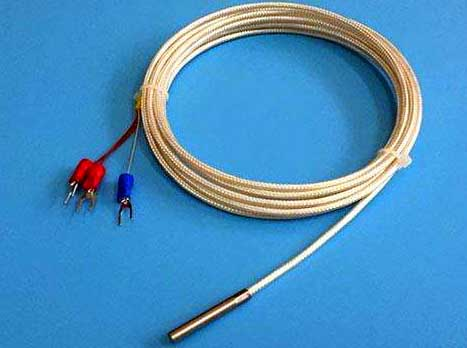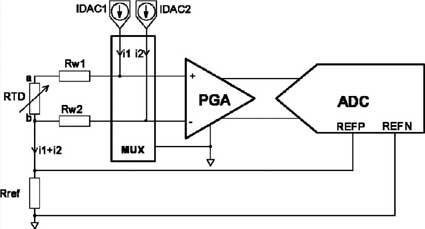﻿ A Precise Thermal Resistance Temperature Measuring Method_

China sensor manufacturers

# A Precise Thermal Resistance Temperature Measuring Method

Summary: This paper introduces a method for accurately measuring the resistance of three-wire thermal resistance by constant voltage partial pressure method. For Pt100 thermal resistance, the detection resolution can reach 0.005W. At the same time, using the calculation method, the obtained temperature accuracy can reach 0.05 °C.
Key words: constant voltage; three-wire system; thermal resistance; accuracyIntroduction
Temperature parameters are one of the most commonly used production process parameters in industrial production. Although there are many different methods for measuring temperature, thermal resistance has become one of the most widely used sensing elements in industrial temperature measurement due to its excellent characteristics. Due to its excellent physical properties, metal platinum makes it the material of choice for the manufacture of thermal resistors. It can be fabricated into a small film form, or wound on ceramic and mica substrates to create a highly stable temperature sensor that can accommodate a variety of complex temperature measurement applications. The Pt100 RTD temperature sensor is the preferred temperature measuring element in the temperature range of -200 ° C to +400 ° C.

At present, in various inspection equipment, such as various inspection thermostats, the equipment is required to provide high-precision temperature indication, which requires high-precision measurement of temperature. For example, in the intelligent two-wire integrated temperature transmitter with Pt100 thermal resistance sensor, high-precision measurement of temperature is also required, so as to ensure the high precision of the transmitter in the full range. In order to eliminate the influence of wire resistance on the measurement, three-wire wire bonding is used in both laboratory and industrial applications to eliminate the influence of wire resistance. This article describes a solution for accurately measuring the resistance of a three-wire thermal resistor, while providing a high-precision temperature conversion method.Three-wire thermal resistance resistance detection circuit
Figure 1 is a detection circuit that uses a constant voltage partial pressure method to accurately measure the resistance of a three-wire thermal resistor. It is actually a detection part of a high-precision temperature transmitter. It uses the AD7705 as an analog-to-digital converter, and the system control CPU uses the P87LPC764. The overall system is a low-power system.

In Figure 1, the resistor body RT is connected to a three-wire system, and RL is a three-wire resistor. Generally, the resistance of each conductor is within 5W. The resistor body and the measuring circuit are connected by three points A, B, and C, and actually form a voltage dividing circuit for the voltage VREF with the resistor R. Under normal circumstances, in order to avoid the measurement error caused by the heating of the resistor body, the current should be less than 3mA. Here, by selecting VREF and R, the current of the driving thermistor is about 0.6 mA. When VREF and R are known, by detecting VAB and VAC, RT can be obtained by calculation to determine the actual temperature. The detection of VAB and VAC is done by the AD7705, which is a dual input channel. The 16-bit sigma-delta A/D converter has an input with a programmable amplifier, which uses 8x gain, channel 1 detects VAC, and channel 2 detects VAB. Referring to Fig. 1, it is easy to obtain the following relations 1 and 2 regarding VAB and VAC, which are actually binary one-time equations in which RT and RL are unknown, and it is easy to solve RT, that is, Equation 3.

VAB = VREF * RL /（R + RT + 2 * RL）（1）
VAC = VREF *（RT + 2 * RL）/（R + RT + 2 * RL）（2）
RT = R *（VAC-2 * VAB）/（VREF-VAC）（3）

When RT is obtained, the actual measured temperature can be indirectly derived. Since the sensor is non-linear, the conventional method often uses a table look-up, fold line, etc. to calculate the temperature. However, these methods are difficult to obtain high accuracy in the limited table space, and are only suitable for industrial occasions with low precision requirements. If the temperature value is derived by using the Rt(t) function formula given in the national standard plus the trial difference method, extremely high precision can be obtained. However, in the previous computer processing methods, due to the complexity of the program, the floating point calculation method is generally avoided. However, the current C51 compiler has been extremely efficient, and the program storage space is not a problem, so I have adopted this high-precision calculation method. The following is a C51 function for calculating RT and measuring temperature. It uses a Pt100 thermal resistance that conforms to the IEC751 standard and TRC=0.003851.
Process_t() /* measures the temperature solution function, known as R, VREF*/
{
Data float vac,vab,rt,rt1,t;
Vab=ad_pro(2); /*A/D conversion for VAB and VAC*/
Rt1=r*(vac-2*vab)/(vref-vac);
/* Calculate the current value of the thermal resistance */
Rt=lubo(rt1); /*sliding weighted filtering*/
t=(rt-100)/0.36;
/*First estimate the current temperature linearly by 400 metrics*/
Do
/* Use the trial and error method to calculate the cycle, the estimated t is the initial value */
{
If(rt<100) /* below zero degrees Celsius */
{
Rt1=100+t*(0.390802-0.0000580195*t-
0.000000000427351*(t-100)*t*t);
}
Else / * Celsius below zero processing * /
{
Rt1=100+t*(0.390802-0.0000580195*t);
}
t=t+(rt-rt1)/0.36;
}
While(((rt-rt1)>0.005)||(rt1-rt)>0.005);
/* Calculated residual is ultimately less than 0.005 ohms*/
Return(t) /* End of trial interval, return floating point temperature value */
}

Calibration of R and VREF
The above solution for measuring temperature is to treat both R and VREF as known parameters. On the surface, they all have nominal values, but their nominal values are all error-prone.
Where R is a high stability metal film resistor with an accuracy of 0.1%, VREF is taken from the LM285, and its factory stability range is 1.235

﻿
﻿